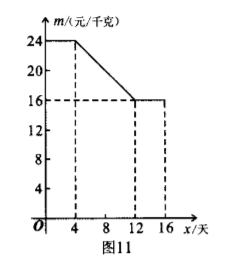(1) 求第 14 天小颌家草䔦的日销售量;
(2) 求当 $4 \leqslant x \leqslant 12$ 时, 草苺价格 $m$ 与 $x$ 之间的函数关系式;
（3）试比较第 8 天与第 10 天的销售金额哪天多?【答案】 解: （1） $\because$ 当 $10 < x \leqslant 16$ 时， $y=-20 x+320$,
$\therefore$ 当 $x=14$ 时, $y=-20 \times 14+320=40$ (千克).
$\therefore$ 第 14 天小颖家草莓的日销售量是 40 千克.
（2）当 $4 \leqslant x \leqslant 12$ 时, 设草苺价格 $m$ 与 $x$ 之间的函数关系式为 $m=k x+b$,
$\because$ 点 $(4,24),(12,16)$ 在 $m=k x+b$ 的图象上,
$\therefore\left\{\begin{array}{l}4 k+b=24, \\ 12 k+b=16 .\end{array}\right.$ 解得 $\left\{\begin{array}{l}k=-1, \\ b=28 .\end{array} \therefore\right.$ 函数关系式为 $m=-x+28 .$
（3） $\because$ 当 $0 \leqslant x \leqslant 10$ 时, $y=12 x, \therefore$ 当 $x=8$ 时, $y=12 \times 8=96$,

$\therefore$ 当 $x=8$ 时, $m=-8+28=20$, 当 $x=10$ 时, $m=-10+28=18$.
$\therefore$ 第 8 天的销售金额为: $96 \times 20=1920$ (元),